# Mho Relay Construction and principle of Operation

A simple form of Mho relay | Admittance or angle admittance relay shown in the figure below: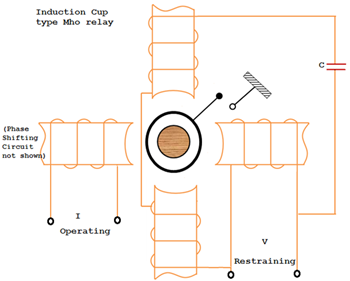It is an electromagnetic induction cup type mho relay.

The torque equation is given by T = K1 VI (Φ – α) – K2V2 – K3

The upper and lower poles are energized by a voltage V to produce a polarizing flux. The capacitor connected in series provides memory action. The left is energized by a current is the operating quantity. The left pole due to current I interacts with the polarized flux due to V produce the operating torque K1VI Cos (Φ – α).

The angle α can be varied by adjusting the resistance in the phase shifting circuit provided on the left pole. The right hand side pole is energized by the voltage and the flux produced by it interacts with the polarizing flux for producing the restraining torque, K2V2.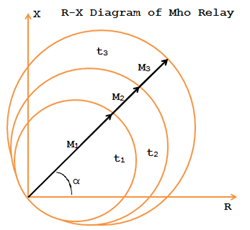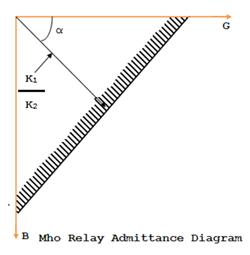A Mho relay measures a component of admittance |Y|  θ. But its characteristic when plotted on the impedance diagram (i.e., R-X diagram) is a circle passing thro’ the origin shown in the fig. It is inherently a directional relay as it detects the fault only in the forward direction. The relay is called Mho Relay because its characteristic is a straight line, when plotted on an admittance diagram (G-B axes i.e., conductance – susceptance axes) as in the figure.

• Read: Directional Over Current Relay
• Read: Solid Sate Relay or Static Relay

## Mho Relay Characteristic Expression

The operating torque for a Mho relay is by V-I element and restraining torque is by voltage element.

Therefore, a Mho relay can be called as a voltage restrained directional relay.

T = K1 VI Cos (Φ – α) –K2V2, neglecting the effect of the spring.

K2V21VI Cos (Φ – α)

K2V 1I Cos (Φ – α)

(V/I Cos (Φ – α)) 1/K2 or (V/I) 1/K2) Cos (Φ – α) or Z 1/K2)Cos (Φ – α)

At balance conditions, the operating torque is equal to restraining torque.

i.e., K1VICos (Φ – α) = K2V2

(I/V)Cos (Φ – α) = (K2K1) = K

(1/Z) = (K / Cos (Φ – α)) = Y

Y = K / Cos (Φ – α) = admittance in mho.

There units of mho relays are used for the protection of a section of the line. I unit is a high speed unit to protect 80% to 90% of the line section. The II unit protects the rest of the line section and its reach extends up to 50% of the adjacent line section. The III unit is meant for backup protection of the adjacent line section. The II and III units operate with a preset time delay, usually 0.2 sec to 0.5 sec and 0.4 sec to 1sec respectively. The time distance characteristic is a stepped one as shown in figure.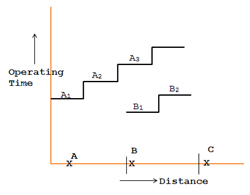## Operation of Impedance and Mho Relay during power Swing

Comparison of Mho characteristic and Impedance Characteristic under power swing conditions AB is the line to be protected. Impedance characteristic relay will trip even for the fault points behind location A which is nothing but a ‘nuisance tripping’.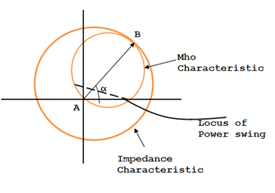Whereas, the Mho characteristics relay requiring a comparatively small area of circle for the line AB, does not sense the faults behind A. Hence many points covered by the impedance characteristic are in the negative torque region of Mho characteristic.

Locus of the power swing, occurring on the long transmission lines during wrong synchronization etc. being a temporary phenomenon is a curve which enters into the operating zone of an impedance relay earlier than that of Mho relay is a curve in the diagram. This is not a desirable one because before allowing the power swing to die down, the transmission line itself is tripped under the grab of protection by the impedance relay. However, in the event of severe and fast occurrence of power swing, the locus may enter the zone of operation of Mho relay which will act and cut off the line which is also undesirable. Hence, in order to avoid this kind of situation, offset Mho relay is used as indicated below.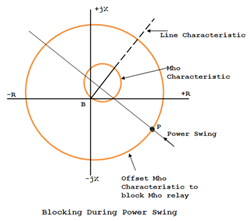During the power swing, the locus of the impedance measured by relay moves along the curve. As soon as it comes within the positive torque region of the offset Mho characteristic (point P), the offset Mho relay acts and blocks the measuring relay for line BC. Therefore the Mho relay does not operate during power swing.
##### Comparison Table
 S. No Type of Relay Operating Torque Element Restraining Torque Element Used for Protection 1 Impedance Relay (Z) Current (I) Voltage (V) Phase faults in medium length lines 2 Reactance  Relay (X) Current (I2) Voltage – Current SinΦ ( V – I sin Φ) Ground faults in short lines 3 Admittance Relay (Y) VI Cos (Φ – α) V Phase faults in long Lines

This post first appeared on My Tech Info, please read the originial post: here